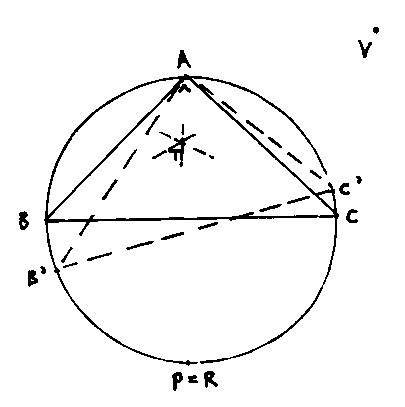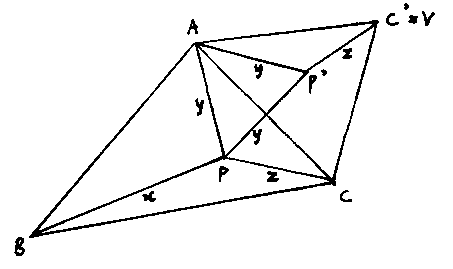Solution Christmas Problem

Let K be a circle with radius 1 and G the closed circular disc with border K. Determine the maximum and minimum values of PA+PB+PC with A,B,C on K, and P in G, if the triangle ABC has area equal to 1.

Find here the names of the winners together with their solutions.

MY OWN SOLUTION:

1) Because the area of triangle ABC is 1, the largest angle is at most 90 degrees, and if the largest is 90 degrees, then the other two must be 45 degrees (else the area is too small).
So we probably get the maximum value if the points are situated as in the picture below. The maximum then is 2+2*sqrt(2).To see that this is true, we think as follows:
If we move C over the circle in the direction away from R, then we have to move B in the direction of R to keep the area of the triangle equal to 1.
Give coordinates A(0,1), R(0,-1),B(-cos(d),-sin(d)), C(cos(e),sin(e)) with small non-negative d,e.
Using the Hesse formula to calculate the distance from a point to a line we get
area ABC = BC*distance(A,BC)/2 = (1/2)(cos(e)+cos(d)-sin(e-d)) = 1.
Triangle ABC has angles (90-d/2+e/2,45-e/2,45+d/2) with d larger than e, so sin(d) is larger than sin(e).
So RB+RC = sqrt(2-2*sin(d))+sqrt(2+2*sin(e)) is smaller than 2*sqrt(2).
For further research, I wrote a computer program (see listing and output ).
The program calculates the value of d for a large amount of values of e. Triangle BCA has angles varying from (45,45,90) to about (33, 73.5,73.5). The optimal point P shifts from the initial position of 270 degrees to a bit more than 290 degrees, and the maximum distance sum decreases from 2+2*sqrt(2)=4.827 to a bit more than 4.3.

{{ 1') Analytical proof for insiders:
From (1/2)(cos(e)+cos(d)-sin(e-d)) = 1 we find by implicit differentiation
d' = ((cos(e-d)+sin(e))/(cos(e-d)-sin(d)); This is larger than 1, so d increases faster than e.
Now suppose that P is (cos(u),sin(u)) with u varying from 3*pi/2 to a bit more.
We have PA+PB+PC = t = 2sin(u/2-pi/4)-2cos(u/2-d/2)+2sin(u/2-e/2).
We find the optimal point with fixed d and e by setting tu=0. Then we get
tan(u/2) = (sin(d/2)-cos(e/2)-wortel(2)/2)/(sin(e/2)+cos(d/2)+wortel(2)/2).
Hence we deduce that the derivative u' of u with respect to e is positive and smaller than 1. The output indicates the same.
Differentiation of t with respect to e yields
t'=tuu'+tdd'+te=(cos(u/2-pi/4)+sin(u/2-d/2)+cos(u/2-e/2))u'- sin(u/2-d/2)d'-cos(u/2-e/2).
Since for u, t en d with values like ours cos(u/2-pi/4) is negative and abs(sin(u/2-d/2)) is greater than abs(cos(u/2-e/2)) and d'-u' greater than 1-u', it follows that t' is smaller than 0, so t is decreasing.
So we find in R the maximum. }}

2) When the distance sum is minimal, P will lie somewhere inside triangle ABC.
We apply a rotation with center A over 60 degrees. see the picture: P becomes P', C becomes C'=V.
So triangle ACV is equilateral.The distance sum is minimal when B,P,P' and V are collinear. So the minimum is BV.
V is the intersection point of the circles with equations
x2+(y-1)2=(cos(e))2+(1-sin(e))2 and
(x-cos(e))2+(y-sin(e))2=(cos(e))2+(1-sin(e))2.
This yields V:(num/(2cos(e)),(num/(2-2sin(e)) with num=cos2(e)+sqrt(cos4(e)+2cos2(e)(2sin2(e) -3sin(e)+1)).
The computer program mentioned above calculates for each triangle BCA the distance BV as well. It turns out that the minimal distance sum increases from 2.7321 for e=0 to 2.8123 for e=24.10.
So the demanded minimum is 2.7321=BV=sqrt(4+2*sqrt(3))=1+sqrt(3), and occurs in the same rectangular triangle with which we began (with P=T=(0,sqrt(3)/3), see the picture).

{{ 2') Analytical proof for insiders:
Let s be the minimal distance sum (like in the problem), so s=BV.
By calculation we find s2 = v = 2(sin2(alpha)+sin2(beta)+sin2(gamma)+ sqrt(3)), with alpha=pi/2-d/2+e/2, beta=pi/4-e/2, gamma=pi/4+d/2.
For (alpha,beta,gamma)=(90,45,45) we find s2=4+2*wortel(3), s=1+wortel(3)=2.73205.
We want to prove that the derivative of v(e) is positive.
Using the formula for d' we found in 1'), we see that this amounts to proving that
(cos(e-d)+sin(e))/(cos(e-d)-sin(d)) is larger than (cos(e)+sin(e-d))/(cos(e)-sin(d-e)).
After a bit of calculation we find that this is true if 2d-e is smaller than pi/2, which holds for our values.
So we find in T the minimum. }}